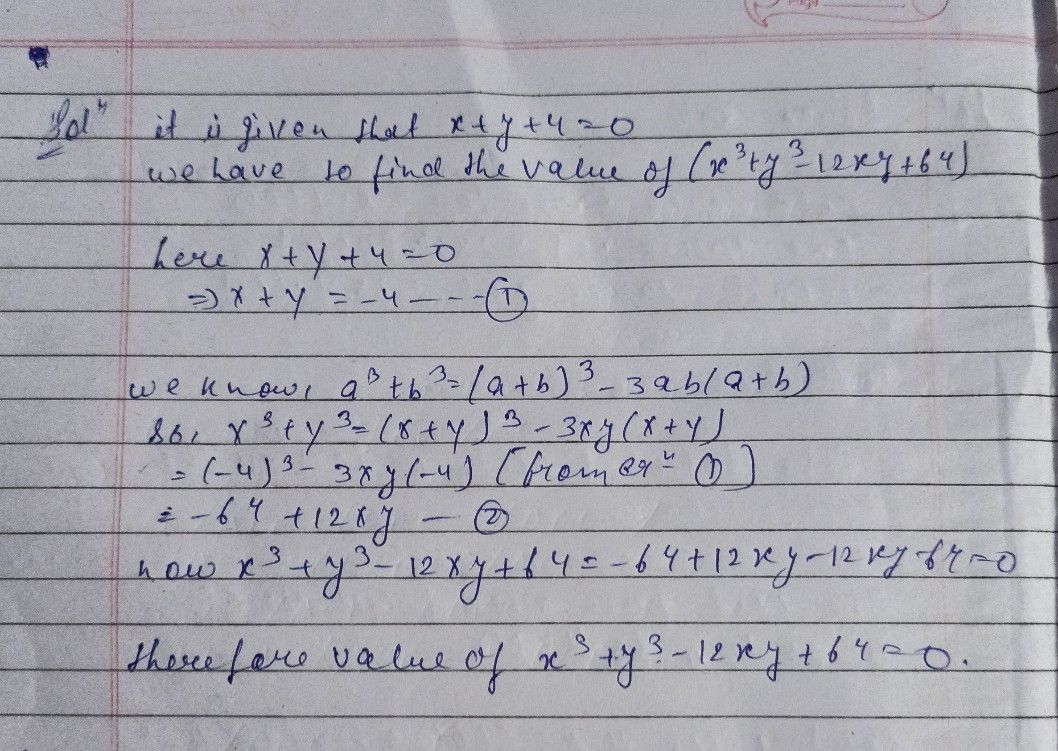Symbol
Problem$2\right)$ Reduce the Quadratic $\left(0$ $6x^{2}+3y^{2}+3z^{2}-4xy-2yz+4xz$ to the sum of squares $onm$
10th-13th grade
Other
Search count: 105
SolutionQanda teacher - pummy